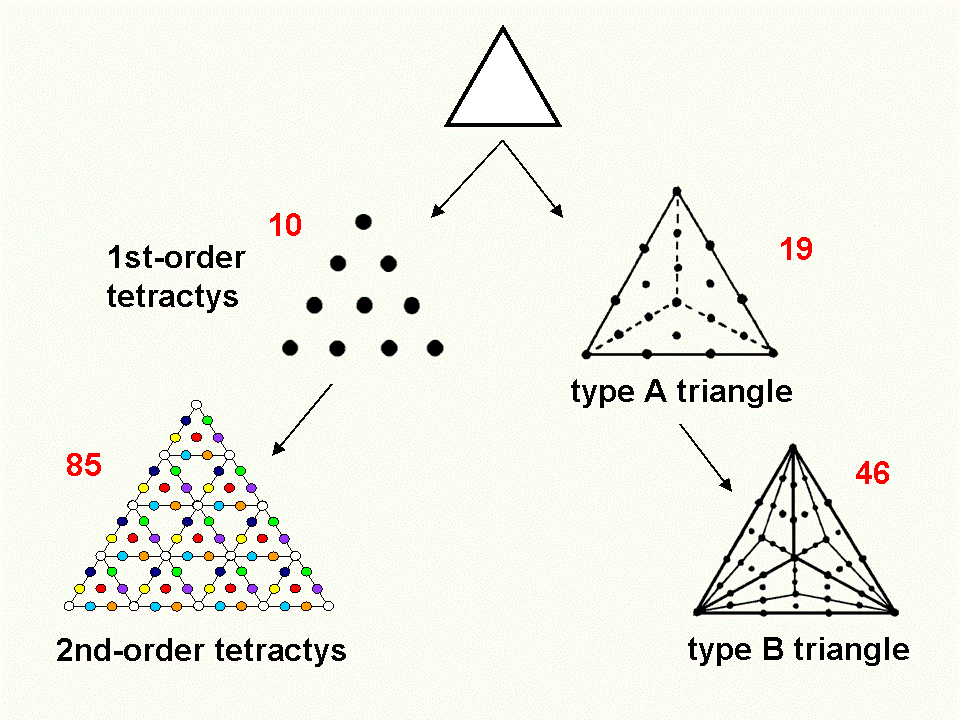<< Previous    1...   6  7    9  10  ...45    Next >>For the purpose of studying sacred geometries, there are two ways of transforming their component triangles: 1. change them into tetractyses ('1st-order tetractyses'), '2nd-order tetractyses,' in which each yod itself becomes a tetractys, and so on; 2. divide each triangle into its sectors, those sectors into three more sectors, etc and then turn each sector into a tetractys. Triangles whose sectors are tetractyses are called 'type A triangles' and triangles whose sectors are divided into three tetractyses are called 'type B triangles,' The numbers in red denote the yod populations of the various, transformed triangles. This division can proceed further into type C triangles, type D triangles, etc. Traditionally, the tetractys has been depicted as having the form of an equilateral triangle. In the context of sacred geometry, however, the component triangles that are its building blocks may be isosceles triangles or, indeed, triangles of any shape. They can all be turned into either tetractyses as triangular arrays of 10 dots or any higher-order tetractys.

 << Previous    1...   6  7    9  10  ...45    Next >>

Home